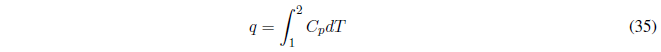We start calculating the work, since P is constant it goes out of the integral.AP constant the heat exchanged is given by the enthalpy change.• If $C_p$ is independent of temperature it can be taken from the integral.
• If we are given as data $C_v$ instead of $C_p$ remember the relationship between both magnitudes, $C_p-C_v=R$.
• The first principle gives us the internal energy change in the process, $\Delta U =q+w$ .
• The enthalpy change coincides with the heat exchanged.$\Delta H =q$